# Unit 1: Getting Ready for Chemistry

 Page 1/19 Date 23.04.2018 Size 1.3 Mb. #45980
Unit 1: Getting Ready for Chemistry

If you can do all the things listed below, you are ready for the Unit 1 test.

Place a checkmark next to each item that you can do! If a sample problem is given, complete it as evidence.
 _____1. I can list five important lab safety rules. 5 important lab safety rules are: 1. wear appropriate personal protection equipment (PPE) 2. follow directions 3. no food or drink in the lab 4. no non-experiment related electronics in the lab 5. no horseplay _____2. I can identify the most common laboratory tools such as: beaker, graduated cylinder, Erlenmeyer flask, scoop, beaker tongs, test tube, test tube rack, test tube holder, crucible tongs, Bunsen burner, striker, stirring rod, funnel, dropper pipette (aka eye dropper) Draw and label as many of the common laboratory tools as you can! _____3. I can determine the independent and dependent variable in a lab experiment. A farmer wants to know what the effect the amount of fertilizer has on the amount of fruit an apple tree produces. What is the independent variable? amount of fertilizer What is the dependent variable? amount of fruit apple tree produces _____4. I can determine the number of significant figures in a measurement. How many significant figures are there in 30.50 cm? 4 How many significant figures are there in 400.0 sec? 4 _____5. I can determine the answer to a math problem to the correct number of significant figures. To the correct number of significant figures, what is the answer to 5.93 mL + 4.6 mL? 10.5 mL To the correct number of significant figures, what is the answer to 5.93 cm * 4.6 cm? 27 cm2 _____6. I can read the meniscus on a graduated cylinder to the correct number of significant figures.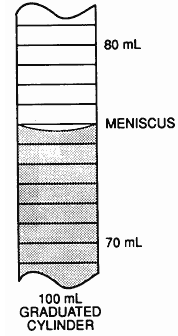The volume is______75.7________ mL. _____7. I can use dimensional analysis to solve math problems. To the correct number of significant figures, determine how many meters there are in 15.4 ft. 4.69 m To the correct number of significant figures, determine how many minutes there are in 2.7 years. 1.4 x 106 min _____8. I can convert numbers into scientific notation from standard notation. Convert 87,394,000,000,000 to scientific notation. 8.7394 x 1013 Convert 0.0000040934 to scientific notation. 4.0934 x 10-6 _____9. I can convert numbers into standard notation from scientific notation. Convert 5.8 x 109 to standard notation. 5,800,000,000 Convert 4.3 x 10-5 to standard notation. 0.000 043 _____10. I can use my calculator to input numbers in scientific notation using the “2nd function & EE keys. Enter the number 5.67 x 1052 on your calculator and show Mrs. S. She’ll initial this box, if you’ve done it correctly! calculator display should read 5.67E52 _____11. I can convert between different metric units by using “King Henry died by drinking chocolate milk”. 9.3 km = ? m 9300 m 39,983 mL = ?kL 0.39983 kL _____12. I can convert between different metric units by using Reference Table C and dimensional analysis. 1.5 x 10-3 km = ? m 1.5 x 106 m 4.67 x 1013 pm = ?dm 467 dm _____13. I can determine which equation to use from Reference Table T by looking at the given information. Which equation would you use to solve the following problem? (Don’t solve it. Just tell me WHICH equation to use.) Problem: How many grams of LiBr (gram-formula mass = 87 g/mol) would 3.5 moles of LiBr be? moles = given mass/gfm _____14. I can solve for “x” when it’s in the denominator of a fraction. What is the volume, in cm3, of 54.6 g of beryllium (density = 1.85 g/cm3) 29.5 cm3 _____15. I can convert oC to degrees kelvin and degrees kelvin to oC. What kelvin temperature is equal to 200oC? 473K What Celsius temperature is equal to 200K? -73oC _____16. Given the symbol I can write the name for any element in Group 1, Group 2, Group 13, Group 14, Group 15, Group 16, Group 17 or Group 18 without using a Periodic Table. Al________aluminum________ Ca________calcium_________ Ne________neon_________ N________nitrogen_________ Na________sodium________ S________sulfur_________ Br_______bromine_________ Ge______germanium______ _____17. Given the symbol or the name, I can determine the Group for any element in Group 1, Group 2, Group 13, Group 14, Group 15, Group 16, Group 17 or Group 18 without using a Periodic Table. Al_____Group 13__________ Ca_____Group 2__________ Ne_____Group 18___________ N______Group 15____________ Na______Group 1_________ S_______Group 16_________ Br_______Group 17________ Ge_______Group 14_______ _____18. I can define gram-formula mass (AKA molar mass). Definition: gram formula mass is the mass of one mole of substance

 _____19.Given the chemical symbol/formula, I can determine how many atoms are present. How many moles of atoms are in N2? 2 What is the total # of moles of atoms in Pb(C2H3O2)2? 15 How many moles of C atoms are in Pb(C2H3O2)2? 4 _____20. I can determine the gram-formula mass for any element or compound. What is the gfm for N2? 28 g/mol What is the gfm for Pb(C2H3O2)2? 325 g/mol _____21. I can define a mole as it pertains to chemistry. Definition: A mole is a unit to measure the amount of substance. One mole of substance is equal to its gfm. It is also equal to 6.02 x 1023 particles. If the substance is a gas at STP, one mole will occupy 22.4 L of volume. _____22. I can find the number of moles of substance if I am given the mass and formula for the substance. 94.3 g is how many moles of NaCl? 1.61 moles _____23. I can determine the percent composition of an element in a compound. What is the percent by mass of Mg in Mg(NO3)2? 18.8% _____24. I can convert between moles and numbers of particles using Avogadro’s number? How many moles of carbon atoms are there in 4.8 x 1024 atoms of C? _____25. I can convert between moles and L (assuming STP). How many L does 4.60 moles of O2 occupy (assuming STP)? 103 L

Unit 2: Introduction to Matter

If you can do all the things listed below, you are ready for the Unit 2 test.

Place a checkmark next to each item that you can do! If a sample problem is given, complete it as evidence.
 _____1. I can still do everything from Unit 1. _____2. I can define the following: atom, element, compound, mixture Definitions: atom – smallest particle of matter that retains the properties of an element element –a substance that cannot be broken down into a simpler substance compound – two or more elements chemically combined in a fixed ratio mixture – two or more substances physically combined in a variable ratio _____3. I can draw particle diagrams to represent an atom, an element, a molecule, a compound, a mixture 2 Atoms of 1 Element 2 Molecules of 1 Compound Mixture of 2 elements Mixture of 2 compounds Mixture of an element and a compound

 _____4. I can classify substances as a pure substance (element or compound) or as a mixture. Put each of the following examples into the correct column. Examples: C12H22O11, NaCl, Fe, salt water, air, CO2, H2, Ar, soda Element Fe H2 Ar Compound C12H22O11 NaCl CO2 Mixture salt water air soda _____5. I can define homogeneous mixture and heterogeneous mixture in terms of particle distribution. Definitions: homogeneous mixture – two or more substances physically combined with a uniform distribution of particles heterogeneous mixture– two or more substances physically combined with a non-uniform (clumpy) distribution of particles _____6. I can give an example of homogeneous and heterogeneous mixtures. Two examples of homogeneous mixtures: a. brass b. a pitcher of Kool-Aid Two examples of heterogeneous mixtures: a. snickers bar b. soil _____7. I can classify a property as physical or chemical. Write “P” for physical or “C” for chemical on the line provided. ___P__copper (II) sulfate is blue. ___C__copper reacts with oxygen. ___P__copper can be made into wire. ___P__copper has a density of 8.96 g/cm3. ___P_ copper melts at 1358K. __C__copper reacts with nitric acid. ___P__copper doesn’t dissolve in water.

 _____8. I can classify a change as physical or chemical. Write “P” for physical or “C” for chemical on the line provided. __P___copper (II) sulfate dissolves in water. __C___copper reacts with oxygen to form solid copper (I) oxide. ___P__solid copper is melted. ___P__a chunk of copper is pounded flat. ___P__copper and zinc are mixed to form brass. __P___a large piece of copper is chopped in half. __C___copper reacts with bromine to form copper (II) bromide. _____9. In a particle diagram, I can distinguish between a physical change and a chemical change.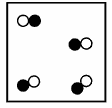Substance A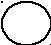Circle the particle diagram that best represents Substance A after a physical change has occurred.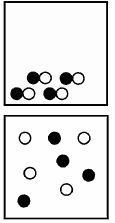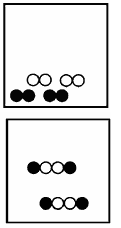_____10. I can define: solute, solvent, solution, and solubility. Definitions: solute – the substance in a mixture that gets dissolved solvent – the substance in a mixture that does the dissolving solution – a homogenous mixture solubility – the amount of solute that will dissolve in a given amount of solvent at a given temperature _____11. I can describe the trend in solubility for solids as the temperature changes. As the temperature increases, the solubility of a solid ___increases____.